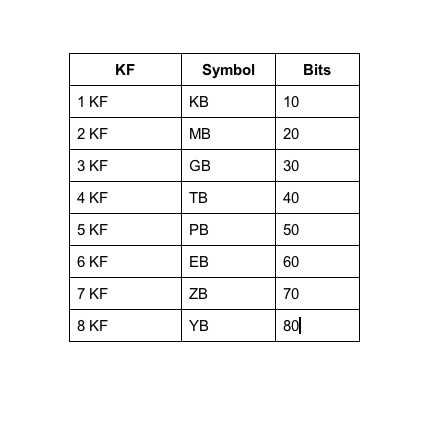# KF Notation for Big Numbers

As storage grows exponentially, it’s getting harder to keep up with the specification of data capacity and other big numbers. Even with kilobytes (KB), megabytes (MB), terabytes (TB), etc., by the time we get to petabytes (PB), exabytes (EB), zettabytes (ZB), and yottabytes (YB), we’ve long lost any sense of relative size.

I’m proposing a notation called K-Factor (KF), which is a size converted to base-1024, i.e., it represents how many factors of 1024, factors of K, are multiplied together. The K-Factor of a value is just the integer part of it’s log-base-1024 value. It’s easier than it sounds.With such a notation in hand, we can cruise on beyond to 9 KF, 10 KF, etc.

Using KF, you might specify a 2-TB disk as a 2-4KF disk and 4 GB of RAM as 4 3KF. Storage space of 16 petabytes would be 16 5KF.

As usual, one would have to recall that a difference between two sizes of 1 KF would mean a factor of 1024 larger, a difference of 2 KF would be roughly a factor of a million (10242), 3 KF approximately a billion, etc.

I have yet to find a similar scheme in the searching I’ve done so far.